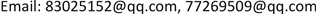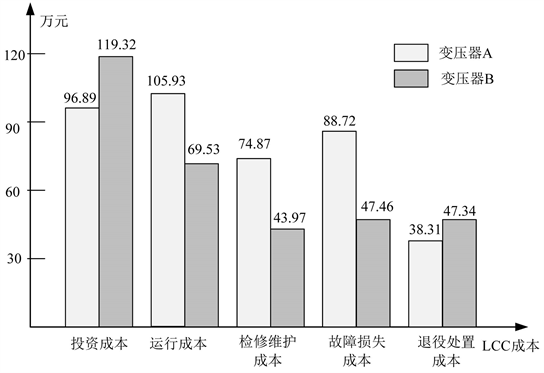﻿ 基于资金时间价值的配电变压器全生命周期模型 Full Life Cycle Model of Distribution Transformer Based on Time Value of Funds

Smart Grid
Vol. 09  No. 03 ( 2019 ), Article ID: 30939 , 9 pages
10.12677/SG.2019.93015

Full Life Cycle Model of Distribution Transformer Based on Time Value of Funds

Ran Hu1, Xifa Wu2

1Shenzhen Power Supply Bureau Co., Ltd., Shenzhen Guangdong

2Shenzhen Xinneng Electric Power Development and Design Institute Co., Ltd., Shenzhen GuangdongReceived: May 31st, 2019; accepted: Jun. 18th, 2019; published: Jun. 25th, 2019ABSTRACT

Because the components of the full life cycle of distribution equipment are dynamic processes, a set of life cycle models for distribution transformers are established to provide a reference for equipment selection in the process of distribution network planning or transformation. Firstly, the main influencing factors of the full life cycle cost of the distribution transformer are fully considered, and the transformer investment phase, operation phase, operation and maintenance phase, failure phase and decommissioning phase are respectively modeled. Then, using the capital time value method, the capital time value model of the transformer life cycle cost is constructed to calculate the full cycle cost of the distribution network transformer throughout the operating period. Finally, the Shenzhen Longgang Bureau distribution network planning project is used as a case deduction. The validity and practicability of the established model are proved by comparing the full life cycle cost of two different types of transformers, and the cost fluctuations of different stages of the distribution transformer are visually reflected by the model simulation results.

Keywords:Distributed Network Transformer, Full Life Cycle Cost, Time Value of Funds, Equipment Selection

1深圳供电局有限公司，广东 深圳

2深圳新能电力开发设计院有限公司，广东 深圳Copyright © 2019 by author(s) and Hans Publishers Inc.1. 引言

20世纪90年代初，我国电力企业开始对资产全寿命周期成本(life cost cycle，LCC)管理方法进行研究，2002年国家电网部分供电公司在工程决策中首次全面推行全寿命周期管理，并取得了预期效果。除此之外，LCC管理在主电网规划、配电网规划、电力工程、高压电力设备选型方面都已经被广泛研究和应用  。

2. 全生命周期成本构成Figure 1. Full life cycle composition

$LC{C}_{变压器}={C}_{I}+{C}_{O}+{C}_{M}+{C}_{F}+{C}_{D}$ (1)

3. 配电网变压器LCC模型

3.1. 变压器投资成本

${C}_{I}={C}_{gm}+{C}_{az}+{C}_{qt}$ (2)

3.2. 变压器运行成本

${C}_{O}={C}_{p}+{C}_{pc}+{C}_{vc}$ (3)

${C}_{PC}=a×{P}_{g}×\left(8760{T}_{S}\right)$ (4)

${T}_{S}=8760×\frac{x}{x+8760/{T}_{R}}$ (5)

${C}_{VC}=a×{E}_{e}$ (6)

${E}_{e}={P}_{L,\mathrm{max}}×{t}_{\mathrm{max}}$ (7)

${P}_{L,\mathrm{max}}=\frac{\left({P}_{A,\mathrm{max}}^{2}+{P}_{R,\mathrm{max}}^{2}\right)×R}{{U}^{2}}=\frac{S×y×R}{{U}^{2}}$ (8)

${T}_{\mathrm{max}}=\frac{E}{{P}_{A,max}}$ (9)

${E}_{\mathrm{max}}=S×y×\left(8760-{T}_{s}\right)$ (10)

${C}_{O}={C}_{P}+a\cdot {P}_{g}\cdot {\left(8760\right)}^{2}\cdot \frac{x}{x+8760/{T}_{R}}+a\cdot {t}_{\mathrm{max}}\cdot \frac{S×y×R}{{U}^{2}}$ (11)

3.3. 变压器检修维护成本

${C}_{M}={C}_{m1}+{C}_{m2}+{C}_{m3}$ (12)

${C}_{m1}={P}_{m1}×{T}_{c}×a+A×\gamma$ (13)

Pm1是由检修造成的单位时间计划失负荷量；Tc为负荷停电持续时间，具体为检修恢复时间、备用电源投入时间或倒闸操作时间等；A为检修过程中开关操作的个数； $\gamma$ 为开关操作费用；a为计划失负荷单位电价。

${C}_{m2}=\underset{i=1}{\overset{K}{\sum }}{a}_{2}{L}_{i}{T}_{i}$ (14)

Li为负荷点i处的平均负荷；Ti为考虑检修设备集合时负荷点i处的年平均停运时间；K为需检修的负荷点个数；a2为随机失负荷单位电价。

${T}_{i}=\underset{j}{\overset{N}{\sum }}\left({{\lambda }^{\prime }}_{j}{{r}^{\prime }}_{j}+{\lambda }_{j}{r}_{j}\right)$ (15)

N为负荷点到电源点的最小路上的设备个数； ${{\lambda }^{\prime }}_{j}$ 为设备j的检修停运率； ${{r}^{\prime }}_{j}$ 为设备j的平均检修持续时间； ${\lambda }_{j}$ 为设备j的故障停运率； ${r}_{j}$ 为设备j的平均故障修复时间。

B为检修设备的集合；Cf,i是设备f选择检修方式i对应的检修费用(该指标根据相对应的设备检修标准得到)。

${C}_{M}=\left({P}_{m1}×{T}_{c}×a+A×\gamma \right)+\left(\underset{i=1}{\overset{K}{\sum }}{a}_{2}{L}_{i}\left[\underset{j}{\overset{N}{\sum }}\left({{\lambda }^{\prime }}_{j}{{r}^{\prime }}_{j}+{\lambda }_{j}{r}_{j}\right)\right]\right)+\underset{f\in B}{\sum }{C}_{f,i}$ (16)

3.4. 变压器故障损失成本

${C}_{F}=\underset{S\in \psi }{\sum }\left[{C}_{f1,s}+{C}_{f2,s}\right]$ (17)

${C}_{f1,s}={P}_{f1,s}×{T}_{C}×{a}_{2}$ (18)

${C}_{f2,s}={\eta }_{S}{C}_{m,s}+\left[1-{\eta }_{S}\right]{C}_{r,s}$ (19)

${C}_{F}=\underset{S\in \psi }{\sum }\left[\left({P}_{f1,s}×{T}_{S}×{a}_{2}\right)+\left({\eta }_{S}{C}_{m,s}+\left[1-{\eta }_{S}\right]{C}_{r,s}\right)\right]$ (20)

3.5. 变压器退役处置成本

${C}_{D}={C}_{bf}-{C}_{cz}$ (21)

4. 基于资金时间价值的变压器LCC模型

$X=Y×\left(X/Y,p,t\right)$ (22)

$\text{LCC}=\underset{t=0}{\overset{T-1}{\sum }}{C}_{t}{\left(\frac{1+r}{1+R}\right)}^{t}$ (23)

$\begin{array}{c}\text{LCC}=\underset{t=0}{\overset{T-1}{\sum }}{\left(\frac{1+r}{1+R}\right)}^{t}\cdot \left[\left({C}_{gm}+{C}_{az}+{C}_{qt}\right)+\left({C}_{P}+a\cdot {P}_{g}{\left(8760\right)}^{2}\frac{x}{x+8760/{T}_{R}}+a\cdot {t}_{\mathrm{max}}\frac{S\cdot y\cdot R}{{U}^{2}}\right)\\ \text{\hspace{0.17em}}\text{\hspace{0.17em}}+\left(\left({P}_{m1}\cdot {T}_{c}\cdot a+A\cdot \gamma \right)+\left(\underset{i=1}{\overset{K}{\sum }}{a}_{2}{L}_{i}\left[\underset{j}{\overset{N}{\sum }}\left({{\lambda }^{\prime }}_{j}{{r}^{\prime }}_{j}+{\lambda }_{j}{r}_{j}\right)\right]\right)+\underset{f\in B}{\sum }{C}_{f,i}\right)\\ \text{\hspace{0.17em}}\text{\hspace{0.17em}}+\left(\underset{S\in \psi }{\sum }\left[\left({P}_{f1,s}\cdot {T}_{S}\cdot {a}_{2}\right)+\left({\eta }_{S}{C}_{m,s}+\left[1-{\eta }_{S}\right]{C}_{r,s}\right)\right]\right)+\left({C}_{bf}-{C}_{cz}\right)\right]\end{array}$ (24)

5. 算例分析Table 2. Cost comparison of various stages of A and B transformersTable 3. LCC comparison of A and B transformers after conversionFigure 2. LCC comparison results of A and B transformers

6. 结论

Full Life Cycle Model of Distribution Transformer Based on Time Value of Funds[J]. 智能电网, 2019, 09(03): 140-148. https://doi.org/10.12677/SG.2019.93015

1. 1. 姚明来, 王艳伟, 刘秦南, 等. 基于全生命周期理论的公共基础设施PPP项目风险动态评价[J]. 工程管理学报, 2017, 31(4): 65-70.

2. 2. 杨磊, 应黎明, 王玉磊. 变电站建设全生命周期成本估算研究[J]. 计算机仿真, 2017, 34(1): 123-128.

3. 3. Mirzadeh, I., Butt, A.A., Toller, S. and Birgisson, B. (2014) Life Cycle Cost Analysis Based on the Fundamental Cost Contributors for Asphalt Pavements. Structure and Infrastructure Engineering, 10, 1638-1647.
https://doi.org/10.1080/15732479.2013.837494

4. 4. 罗晓初, 李乐, 魏志连, 等. 全寿命周期成本理论在配电变压器改造投资决策中的应用[J]. 电网技术, 2011, 35(2): 207-211．

5. 5. 夏成军, 邱桂华, 黄冬燕, 等. 电力变压器全寿命周期成本模型及灵敏度分析[J]. 华东电力, 2012, 40(1): 26-30.

6. 6. 李娜, 王晓亮, 李明, 等. 电力变压器全寿命周期成本建模及其灵敏度分析[J]. 山东电力技术, 2015, 42(11): 36-39.

7. 7. 尹来宾, 范春菊, 崔新奇, 等. 基于全生命周期费用(LCC)的配电装置改造的研究[J]. 电力系统保护与控制, 2011, 39(6): 61-65+70.

8. 8. 陈敏, 佘双翔, 刘小松, 等. 基于LCC的海上风电场主变压器冗余配置经济性对比与分析[J]. 电力系统自动化, 2015(14): 168-174.

9. 9. 李存斌, 庆格夫, 陆龚曙, 等. 基于全生命周期成本的配电网变压器选型系统动力学模型[J]. 现代电力, 2014, 31(3): 86-90.

10. 10. 马健. 基于全生命周期成本理论的220kV电力变压器采购研究[D]: [硕士学位论文]. 广州: 华南理工大学, 2017.